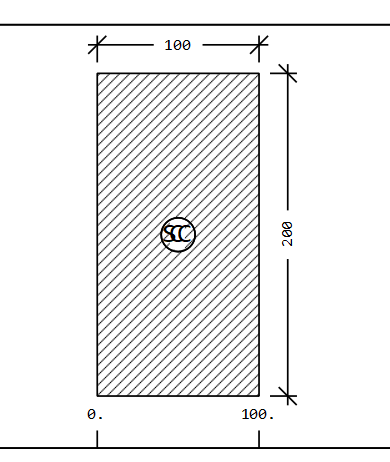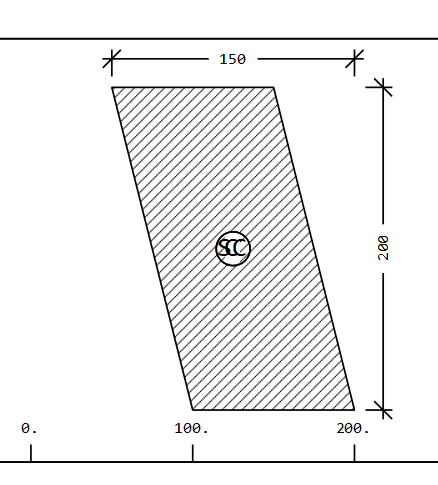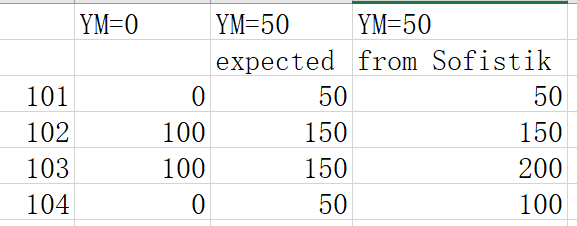# Offset of all cross section ordinates in Aqua

I draw a rectangle in Aqua w/o YM, the result is a rectangle.With YM, I expect a shifted rectangle in Y direction, but the result is a shifted parallelogram.POLY TYPE O MNO 1 YM 50
VERT NO Y Z REFP
101 0 0
102 ‘=B’ 0 101
103 0 ‘=H’ 102
104 ‘=-B’ 0 103

According to Aqua:

YM and ZM can Offset of all cross section ordinates

1. Why only point 103, 104 gets additional 50 in Y direction?
This is the Y coordinates, I expect they should be in the middle column (expected)2. If I want to shift a shape using YM, ZM, how to do it in Aqua?

The dat is attached

RECT.dat (475 Bytes)

Hello,

The parameter YM or ZM shifts the coordinate system.
The coordinates of VERT are relativ to this new coordinate system. Everytime a point refers to the coordinate system, the new value YM or ZM is used. If you refer to a polygon point, nothing changes. This means Y=0 is actually Y=YM.
For example:

``````SECT	1
POLY	TYPE	O	MNO 1   YM  50
VERT	NO	Y	 Z      REFP
101	0	 0
102	'=B'     0      101
103	0	 '=H'   102
104	'=-B'    0      103
``````

This could als be written as

``````SECT	2
POLY	TYPE	O	MNO 1
VERT	NO	Y	 Z	 REFP
101	50	 0
102	'=B'     0       101
103	50	 '=H'    102
104	'=-B'    0       103
``````

And what do you expect from shifting the cross section?
If you want to move the crosssection, just add 50 to the Y-Values.

Best regards,
Frederik Höller